Research papers and code for "Mark Burgin":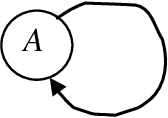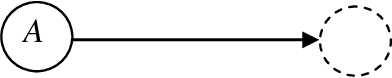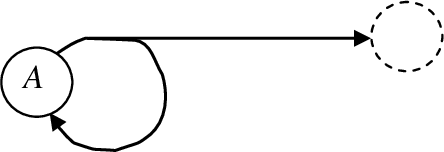Multi-agent approach has become popular in computer science and technology. However, the conventional models of multi-agent and multicomponent systems implicitly or explicitly assume existence of absolute time or even do not include time in the set of defining parameters. At the same time, it is proved theoretically and validated experimentally that there are different times and time scales in a variety of real systems - physical, chemical, biological, social, informational, etc. Thus, the goal of this work is construction of a multi-agent multicomponent system models with concurrency of processes and diversity of actions. To achieve this goal, a mathematical system actor model is elaborated and its properties are studied.

Click to Read Paper and Get Code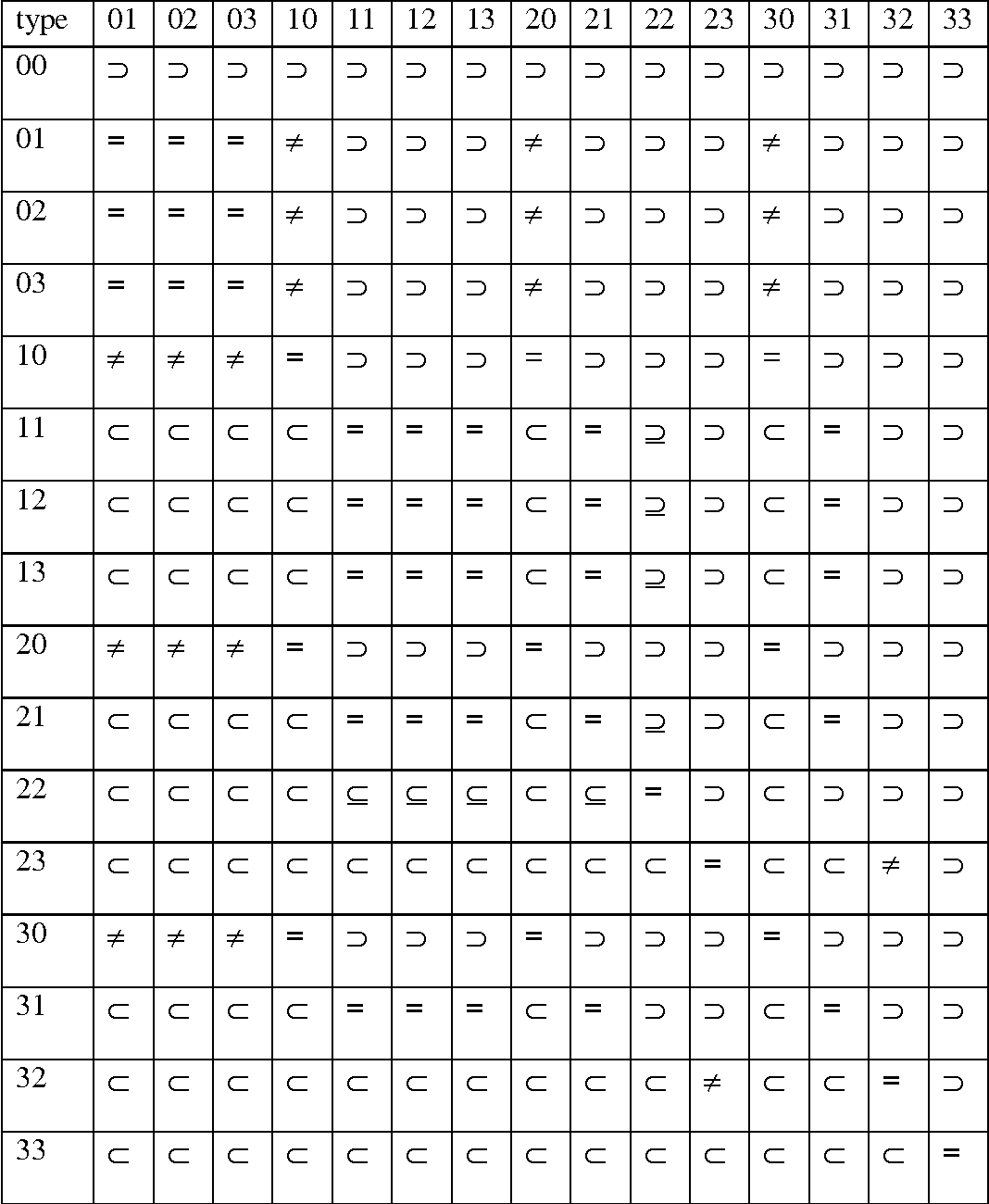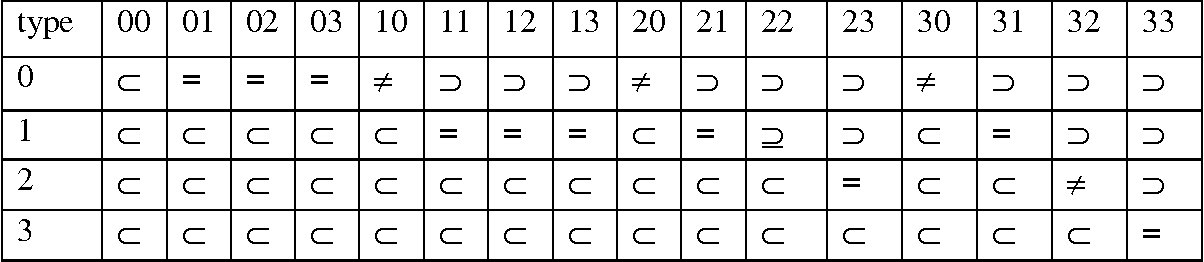A practical tool for natural language modeling and development of human-machine interaction is developed in the context of formal grammars and languages. A new type of formal grammars, called grammars with prohibition, is introduced. Grammars with prohibition provide more powerful tools for natural language generation and better describe processes of language learning than the conventional formal grammars. Here we study relations between languages generated by different grammars with prohibition based on conventional types of formal grammars such as context-free or context sensitive grammars. Besides, we compare languages generated by different grammars with prohibition and languages generated by conventional formal grammars. In particular, it is demonstrated that they have essentially higher computational power and expressive possibilities in comparison with the conventional formal grammars. Thus, while conventional formal grammars are recursive and subrecursive algorithms, many classes of grammars with prohibition are superrecursive algorithms. Results presented in this work are aimed at the development of human-machine interaction, modeling natural languages, empowerment of programming languages, computer simulation, better software systems, and theory of recursion.

* 2 tables
Click to Read Paper and Get Code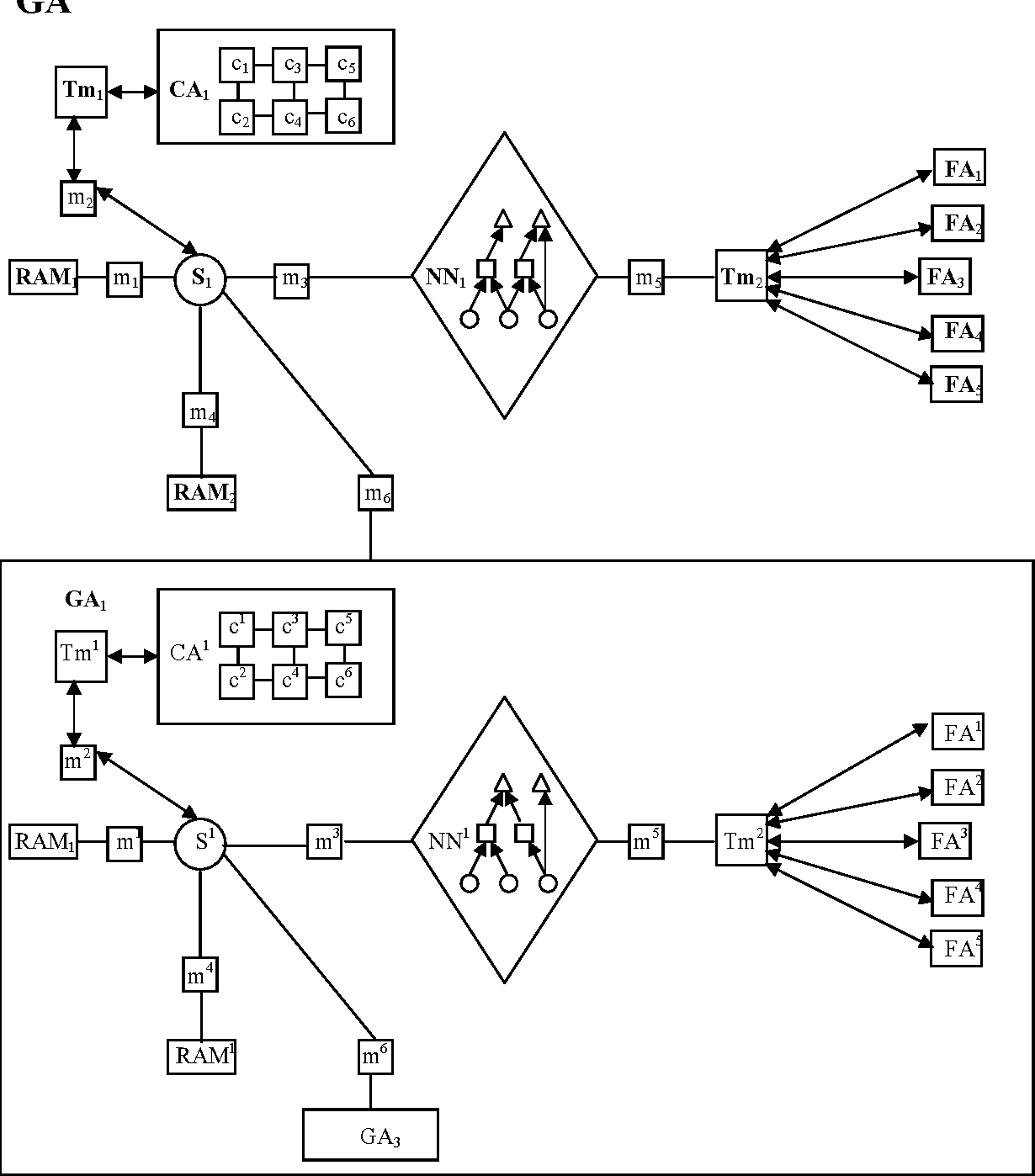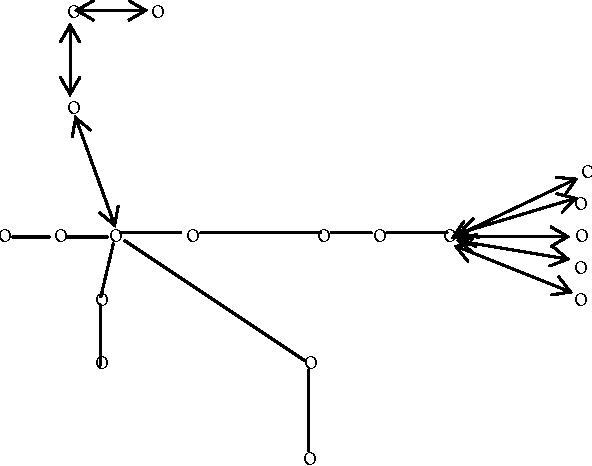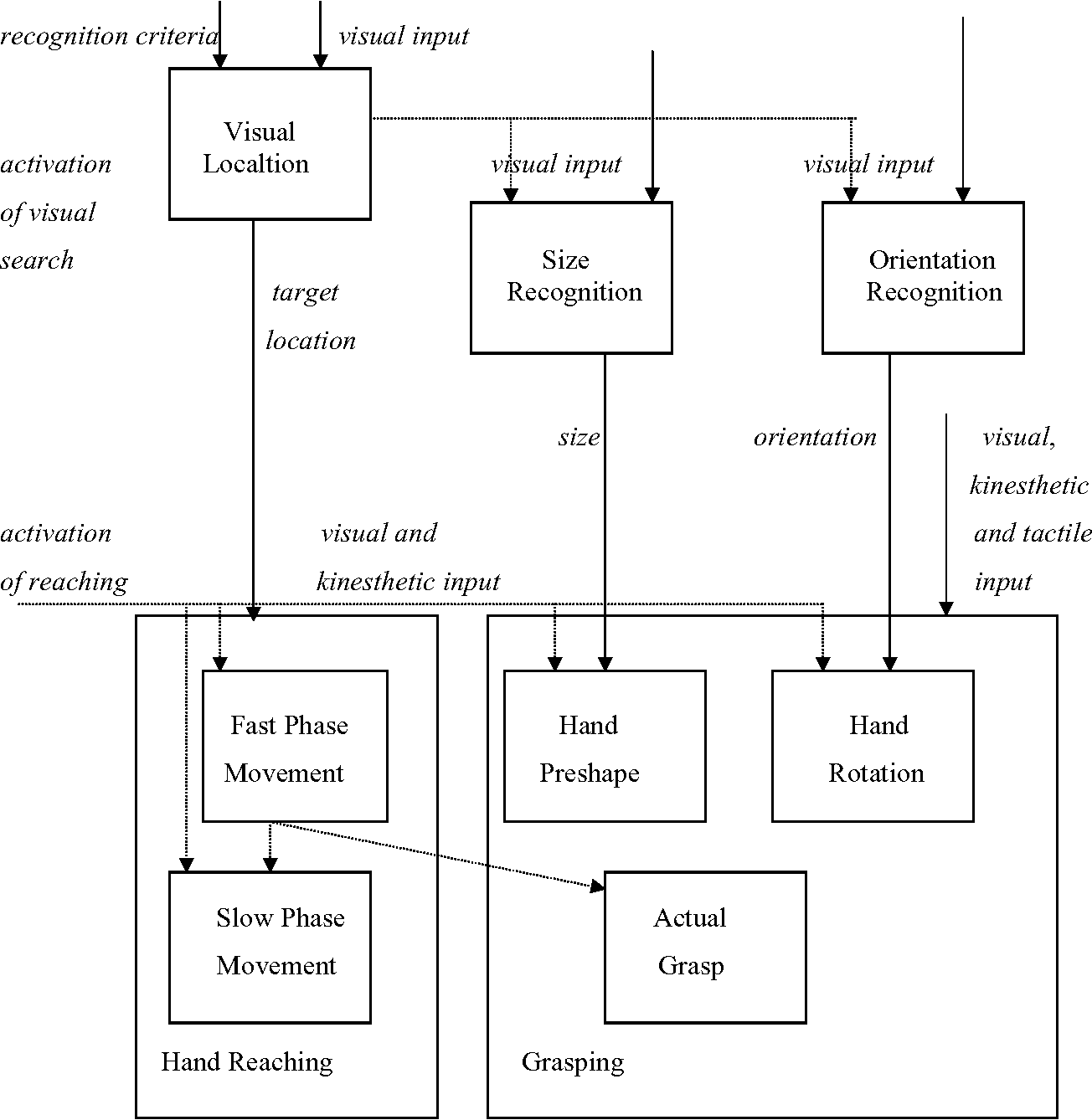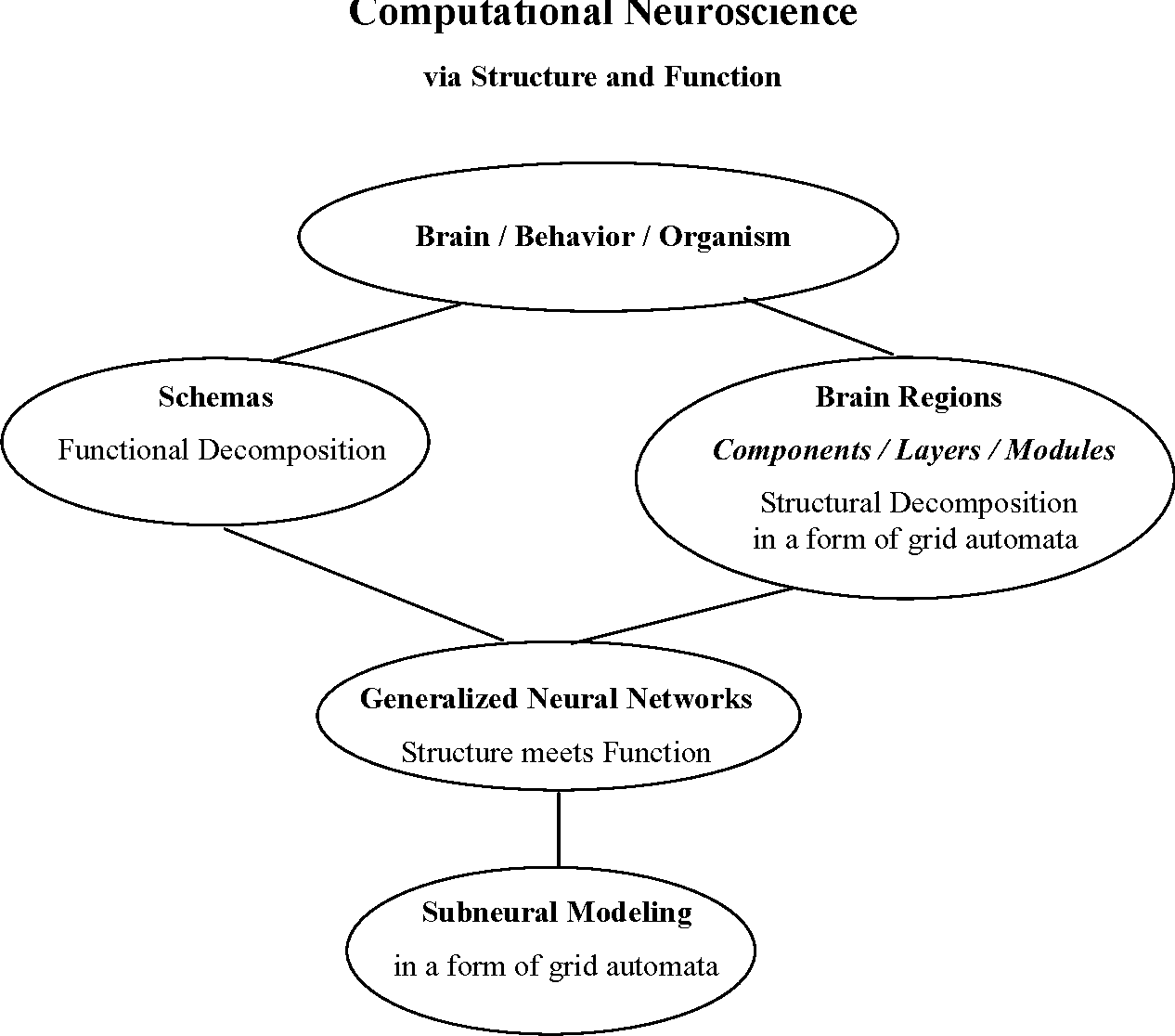In this paper, a mathematical schema theory is developed. This theory has three roots: brain theory schemas, grid automata, and block-shemas. In Section 2 of this paper, elements of the theory of grid automata necessary for the mathematical schema theory are presented. In Section 3, elements of brain theory necessary for the mathematical schema theory are presented. In Section 4, other types of schemas are considered. In Section 5, the mathematical schema theory is developed. The achieved level of schema representation allows one to model by mathematical tools virtually any type of schemas considered before, including schemas in neurophisiology, psychology, computer science, Internet technology, databases, logic, and mathematics.

Click to Read Paper and Get Code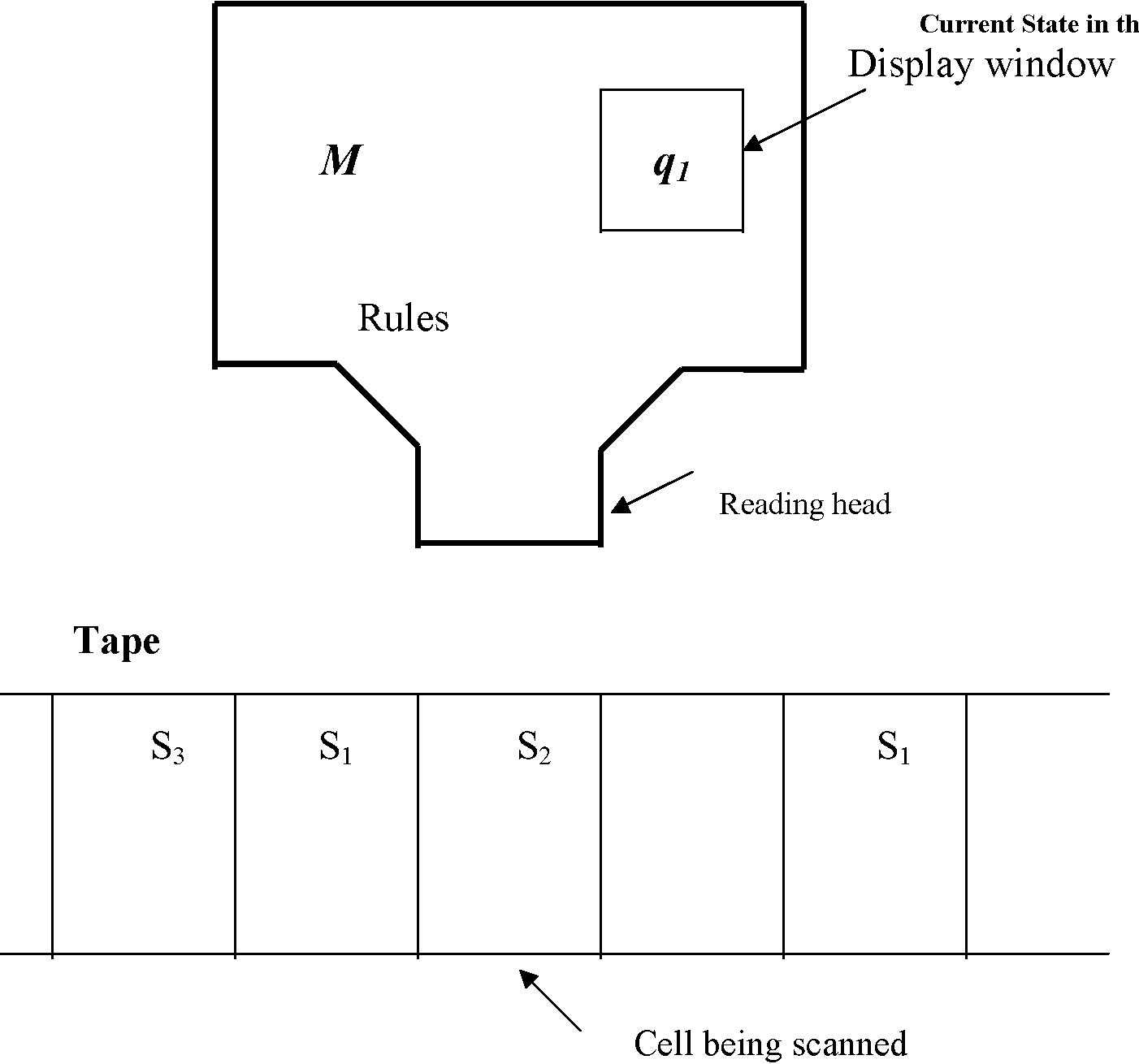The essay consists of three parts. In the first part, it is explained how theory of algorithms and computations evaluates the contemporary situation with computers and global networks. In the second part, it is demonstrated what new perspectives this theory opens through its new direction that is called theory of super-recursive algorithms. These algorithms have much higher computing power than conventional algorithmic schemes. In the third part, we explicate how realization of what this theory suggests might influence life of people in future. It is demonstrated that now the theory is far ahead computing practice and practice has to catch up with the theory. We conclude with a comparison of different approaches to the development of information technology.

Click to Read Paper and Get Code
Many mathematical models utilize limit processes. Continuous functions and the calculus, differential equations and topology, all are based on limits and continuity. However, when we perform measurements and computations, we can achieve only approximate results. In some cases, this discrepancy between theoretical schemes and practical actions changes drastically outcomes of a research and decision-making resulting in uncertainty of knowledge. In the paper, a mathematical approach to such kind of uncertainty, which emerges in computation and measurement, is suggested on the base of the concept of a fuzzy limit. A mathematical technique is developed for differential models with uncertainty. To take into account the intrinsic uncertainty of a model, it is suggested to use fuzzy derivatives instead of conventional derivatives of functions in this model.

Click to Read Paper and Get Code
One of the roots of evolutionary computation was the idea of Turing about unorganized machines. The goal of this work is the development of foundations for evolutionary computations, connecting Turing's ideas and the contemporary state of art in evolutionary computations. To achieve this goal, we develop a general approach to evolutionary processes in the computational context, building mathematical models of computational systems, functioning of which is based on evolutionary processes, and studying properties of such systems. Operations with evolutionary machines are described and it is explored when definite classes of evolutionary machines are closed with respect to basic operations with these machines. We also study such properties as linguistic and functional equivalence of evolutionary machines and their classes, as well as computational power of evolutionary machines and their classes, comparing of evolutionary machines to conventional automata, such as finite automata or Turing machines.

Click to Read Paper and Get Code
Evolutionary processes proved very useful for solving optimization problems. In this work, we build a formalization of the notion of cooperation and competition of multiple systems working toward a common optimization goal of the population using evolutionary computation techniques. It is justified that evolutionary algorithms are more expressive than conventional recursive algorithms. Three subclasses of evolutionary algorithms are proposed here: bounded finite, unbounded finite and infinite types. Some results on completeness, optimality and search decidability for the above classes are presented. A natural extension of Evolutionary Turing Machine model developed in this paper allows one to mathematically represent and study properties of cooperation and competition in a population of optimized species.

Click to Read Paper and Get Code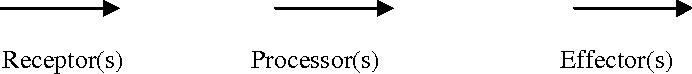Agents and agent systems are becoming more and more important in the development of a variety of fields such as ubiquitous computing, ambient intelligence, autonomous computing, intelligent systems and intelligent robotics. The need for improvement of our basic knowledge on agents is very essential. We take a systematic approach and present extended classification of artificial agents which can be useful for understanding of what artificial agents are and what they can be in the future. The aim of this classification is to give us insights in what kind of agents can be created and what type of problems demand a specific kind of agents for their solution.

Click to Read Paper and Get Code